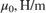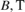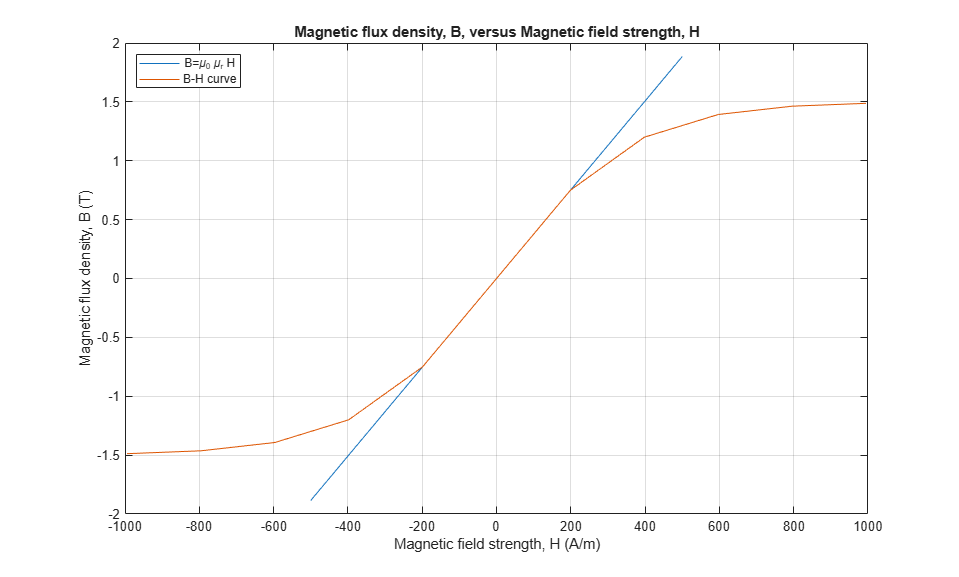# Nonlinear Inductor Characteristics

This example shows a comparison of nonlinear inductor behavior for different parameterizations. Starting with fundamental parameter values, the parameters for linear and nonlinear representations are derived. These parameters are then used in a Simscape™ model and the simulation outputs compared.

### Specification of Parameters

Fundamental parameter values used as the basis for subsequent calculations:

• Permeability of free space,• Relative permeability of core,• Number of winding turns,• Effective magnetic core length,• Effective magnetic core cross-sectional area,• Core saturation begins,• Core fully saturated,### Calculate Magnetic Flux Density and Magnetic Field Strength Data

Where:

• Magnetic flux density,• Magnetic field strength,Linear representation:

•Nonlinear representation (including coefficient, a):

•### Display Magnetic Flux Density Versus Magnetic Field Strength

The linear and nonlinear representations can be overlaid.### Calculate Magnetic Flux and Current Data

Where:

• Magnetic flux,• Current,Linear representation:

••Nonlinear representation:

••### Display Magnetic Flux Versus Current

The linear and nonlinear representations can be overlaid.### Use Parameters in Simscape Model

The parameters calculated can now be used in a Simscape model. Once simulated, the model is set to create a Simscape logging variable, simlog.### Conclusion

The state variable for all representations is magnetic flux,. Current, I, and magnetic flux,, can be obtained from the Simscape logging variable, simlog, for each representation. Overlaying the simulation results from the representations permits direct comparison.